### IMO Shortlist 1996 problem A7

Kvaliteta:
Avg: 0,0
Težina:
Avg: 9,0
Let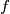$f$ be a function from the set of real numbers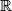$\mathbb{R}$ into itself such for all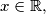$x \in \mathbb{R},$ we have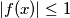$|f(x)| \leq 1$ and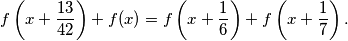Prove that$f$ is a periodic function (that is, there exists a non-zero real number$c$ such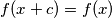$f(x+c) = f(x)$ for all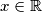$x \in \mathbb{R}$).
Izvor: Međunarodna matematička olimpijada, shortlist 1996